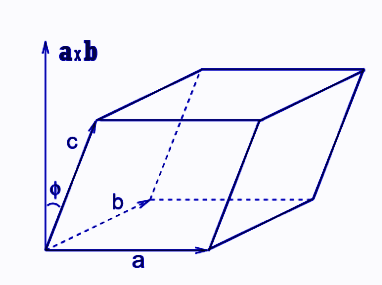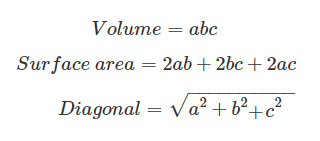# Parallelepiped

Parallelepiped is a 3-D shape whose faces are all parallelograms. It is obtained from a Greek word which means ‘an object having parallel plane’. Basically, it is formed by six parallelogram sides to result in a three-dimensional figure or a Prism, which has a parallelogram base. We can define it as a polyhedron, where three pairs of parallel faces are joined together to form a three-dimensional shape, having six faces. The cube, cuboid and rhomboid are its three special cases. The rectangular parallelepiped has all the faces in a rectangular shape.

## Properties of Parallelepiped

• It is a three-dimensional solid figure.
• Any three faces can be viewed at the same time.
• It has three sets of four parallel edges and the edges within each set have equal measurement of length.
• The diagonal of each face is called face diagonal.
• On observing from outside, each face seems the mirror image of the opposite face.
• It signifies a Prism of parallelogram base.
• It is a polyhedron of six faces.
• The three pairs of parallel faces form a hexahedron.## Volume and Surface Area Formula

For a given parallelepiped, let S is the area of the bottom face and H is the height, then the volume formula is given by;

 V = S × H

Since the base of parallelepiped is in the shape of a parallelogram, therefore we can use the formula for the area of the parallelogram to find the base area.

• Area of Parallelogram = Length × Height

Lateral Surface Area (LSA): Product of perimeter of the base and the height of the 6 parallelograms faced prism.

 LSA = Perimeter of the base × Height

Total Surface Area (TSA): Addition of Lateral surface area and twice the base area

 TSA = LSA + 2 Base Area

## Rectangular Parallelepiped

When all the six faces of parallelepiped are in a rectangular shape, then it is considered a rectangular parallelepiped. It is a three-dimensional box-shaped structure. The length of all the parallel edges here are equal. The base of the prism here is rectangular in shape. A common example you can see in real life is the shoe box, which has a rectangular shape.

 Volume of Rectangular Parallelepiped = Surface Area × Height

Here, the surface area is equal to the area of rectangle = Length × Width

Therefore, the volume becomes;

V = Length × Width × Height

So, if we know these three dimensions of the rectangular box, we can find its volume. Suppose, length = a, width = b and height = c, we can write the formula of volume, surface area and length of the diagonal of the rectangular box as;### Example

The base face of a parallelepiped has opposite sides measuring 5 inches and 10 inches. The height of the parallelepiped is 4 inches. Find the cost of painting its walls from outside at a cost of INR 1.5 per square inch.

Solution: We need to find the lateral surface area first, therefore;

LSA = Perimeter of base × height

LSA = 2 (5 + 10) × 6

LSA = 180 sq.inch

Cost of painting = Lateral surface area × cost per square inch

Cost of painting the walls = 180 × 1.5 = Rs.270/-

## Frequently Asked Questions on Parallelepiped

Q1

### What is the volume of a parallelepiped?

The volume of a parallelepiped is equal to the product of its surface area and height.

Q2

### What is a rectangular parallelepiped?

A rectangular parallelepiped is a three-dimensional structure whose all the six faces are in a rectangular shape and the length of the parallel edges are equal.

Q3

### What is the shape of parallelepiped?

A parallelepiped is a three-dimensional figure made of six parallelograms. It has straight edges and flat faces. The shape is related to parallelogram.

Q4

### What is the area of parallelepiped?

The surface area of parallelepiped is equal to the sum of the lateral surface area and twice the base area.
Lateral surface area (LSA) is equal to the product perimeter of the base and height of the parallelepiped.
Total surface area = LSA + 2 Base Area = (Perimeter of the base x height) + 2 Base area